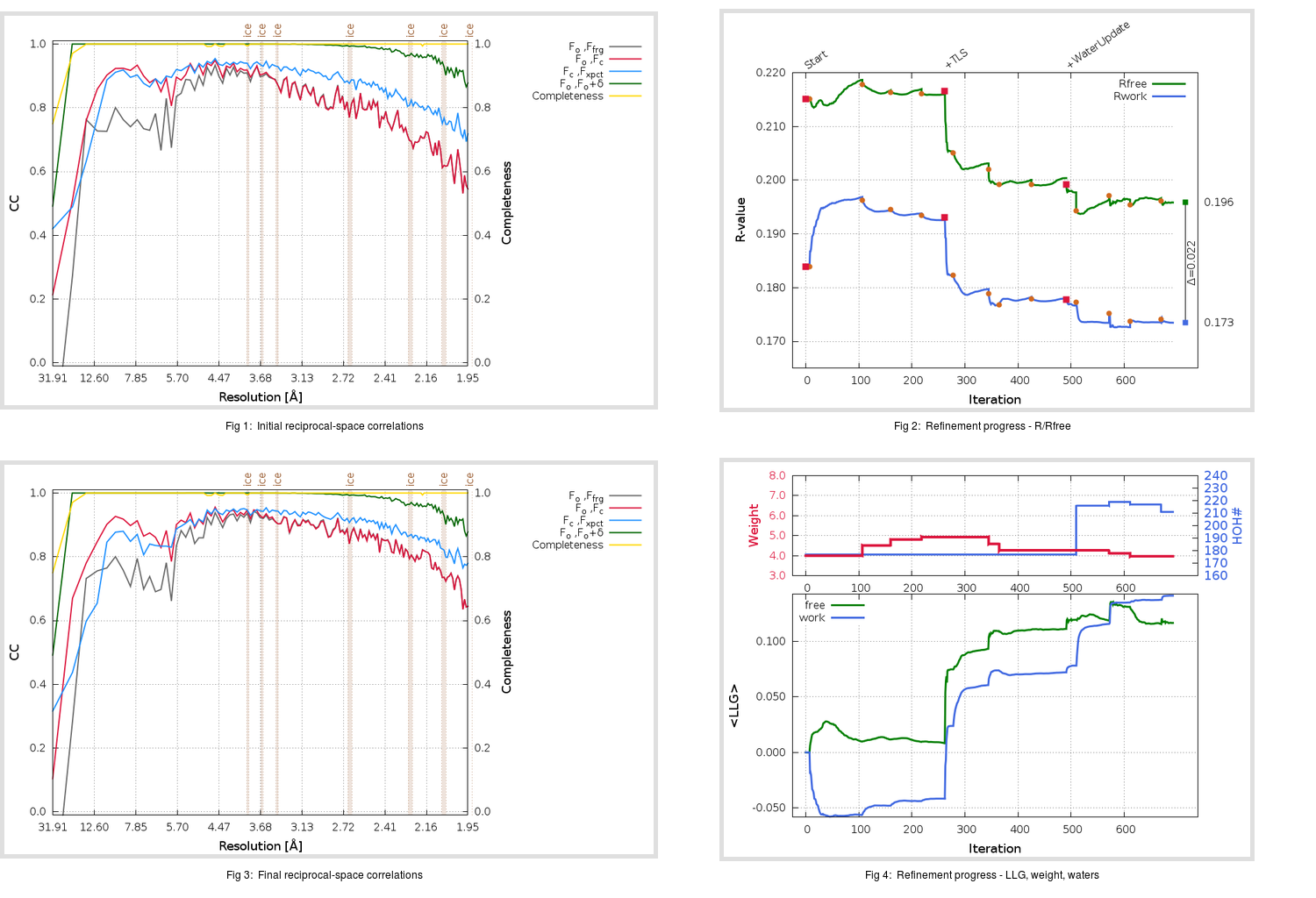Content:

```    Diffraction limits & principal axes of ellipsoid fitted to diffraction cut-off surface:
1.942         0.9282   0.0000   0.3721       a* - 0.030 c*
1.912         0.0000   1.0000   0.0000       b*
1.873        -0.3721   0.0000   0.9282      -0.578 a* + 0.816 c*
```

## Deposited

` `
 Date deposited Date data collection Resolution R, Rfree 20200215 20200201 1.95 0.1779 0.2191

Molprobity (CCP4 7.0 version) summary:

```Ramachandran outliers =   0.33 %
favored =  95.32 %
Rotamer outliers      =   3.46 %
C-beta deviations     =     1
Clashscore            =   2.99
RMS(bonds)            =   0.0146
RMS(angles)           =   1.95
MolProbity score      =   1.82
Resolution            =   1.95
R-work                =   0.1779
R-free                =   0.2191
```

```Number of waters      =   177

<B> (all atoms) =   39.73 ( sd =   15.43 ) for       2565 non-hydrogen atoms
<B>   (protein) =   39.00 ( sd =   15.46 ) for       2341 non-hydrogen atoms
<B>     (water) =   47.49 ( sd =   12.87 ) for        177 non-hydrogen atoms
<B>    (others) =   47.24 ( sd =   12.35 ) for         47 non-hydrogen atoms

B min/max       (all non-hydrogen atoms) =   17.17 /  113.17
B min/max   (protein non-hydrogen atoms) =   17.17 /  113.17
B min/max     (water non-hydrogen atoms) =   19.59 /   92.40
B min/max     (other non-hydrogen atoms) =   30.60 /   81.63
```

## BUSTER (re-)refinement

` `

Molprobity (CCP4 7.0 version) summary:

```Ramachandran outliers =   0.00 %
favored =  98.33 %
Rotamer outliers      =   3.08 %
C-beta deviations     =     0
Clashscore            =   1.71
RMS(bonds)            =   0.0114
RMS(angles)           =   1.53
MolProbity score      =   1.30
Resolution            =   1.95
R-work                =   0.1735
R-free                =   0.1959
```

```Number of waters      =   211

<B> (all atoms) =   43.62 ( sd =   14.23 ) for       2599 non-hydrogen atoms
<B>   (protein) =   42.46 ( sd =   13.77 ) for       2341 non-hydrogen atoms
<B>     (water) =   55.44 ( sd =   14.65 ) for        211 non-hydrogen atoms
<B>    (others) =   48.47 ( sd =    9.07 ) for         47 non-hydrogen atoms

B min/max       (all non-hydrogen atoms) =   20.02 /   92.64
B min/max   (protein non-hydrogen atoms) =   20.02 /   92.64
B min/max     (water non-hydrogen atoms) =   22.96 /   86.87
B min/max     (other non-hydrogen atoms) =   38.19 /   74.33
```

Refinement progression:Results:

` `
 File Remark 6Y2F_aB_refine.01_03_refine.pdb.gz exact refinement commands are in header 6Y2F_aB_refine.01_03_refine.mtz.gz including original deposited data and several re-refinement map coefficients 6Y2F_aB_refine.01_03_BUSTER_model.cif.gz including any non-standard compound restraints 6Y2F_aB_refine.01_03_BUSTER_refln.cif.gz